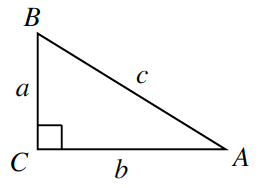### Home > INT3 > Chapter 12 > Lesson 12.1.4 > Problem12-65

12-65.

Using the triangle at right, determine:

This problem is similar to problem 12-58. Refer back to that problem if you need some help.

1. $\operatorname { tan } ( A )$

Remember that $\tan(x)$ is equal to opposite over adjacent.

$\frac{a}{b}$

1. $\operatorname { cot } ( A )$

1. $\operatorname { tan } ( B )$

This is related to part (a). How?